# Electric Field & the Movement of Charge

An error occurred trying to load this video.

Try refreshing the page, or contact customer support.

Coming up next: Strength of an Electric Field & Coulomb's Law

### You're on a roll. Keep up the good work!

Replay
Your next lesson will play in 10 seconds
• 0:01 What Is an Electric Field?
• 1:41 Electric Field and Motion
• 2:31 Equation
• 3:37 Example Calculation
• 4:55 Lesson Summary

Want to watch this again later?

Timeline
Autoplay
Autoplay
Speed

#### Recommended Lessons and Courses for You

Lesson Transcript
Instructor: David Wood

David has taught Honors Physics, AP Physics, IB Physics and general science courses. He has a Masters in Education, and a Bachelors in Physics.

After watching this video, you will be able to explain what an electric field is, draw electric field lines and vectors for charged particles, and complete calculations involving electric fields. A short quiz will follow.

## What is an Electric Field?

Charge is a property of a particle and can be positive or negative. For example, an electron has a charge of negative 1.6 x 10^-19 coulombs. Coulomb is the unit of charge. Charges create electric fields, and the electric field created by one charge will cause other charges nearby to feel a force: opposite charges attract, and similar charges repel.

An electric field is the electric force per unit charge. Or in other words, it's the force that a positive 1 coulomb test charge would feel. A test charge is just a charge you use to do a test, an experiment. So in other words, to figure out the electric field strength at a location, all you have to do is take a charge of +1 coulomb, put it at that position and measure the force it feels. Electric field is measured in newtons per coulomb (N/C).

Electric fields can be represented diagrammatically using either electric field lines or electric field vectors. For example, the electric field around a positive point charge looks like this.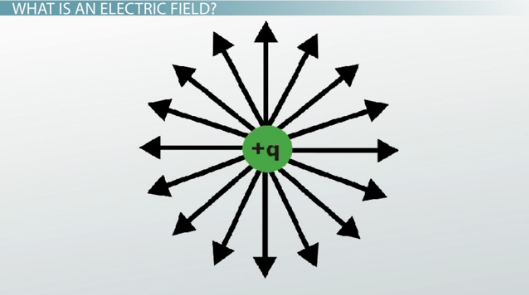Vectors tell you the strength of the field at the point where the arrow begins. The longer the arrow, the stronger the field. With electric field lines, the closer the lines are together, the stronger the field. Notice that the lines and arrows point away from the positive charge -- this is because a positive test charge would be repelled, would be pushed away from this positive charge.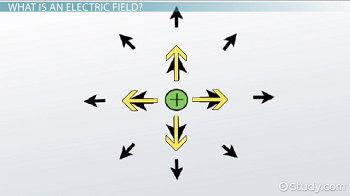Here are the field vectors and lines for a negative charge. They look similar, but the arrows point towards the negative charge. That's because a positive charge would be attracted to it.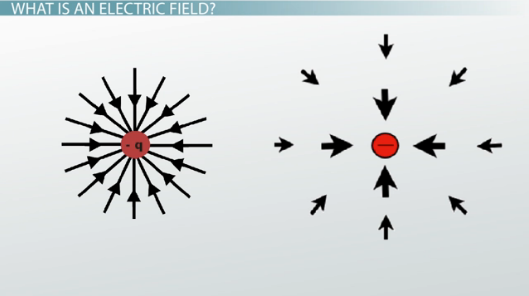## Electric Field and Motion

One important thing to be careful about is that you don't confuse electric fields with the motion of charges. If I release a positive test charge from rest at a position, the charge will indeed follow the electric field lines. But what the field lines really tell you is the force that the charge is feeling. With an initial velocity of zero, any object will move in the direction of a force. But that need not always be the case.

For example, if your positive test charge is moving north, and you enter a field pointing west, the charge will curve in a circle vaguely northeast. Eventually it will follow the field lines and move west, but at first it could go in any direction depending on its initial velocity.

A net force applied to an object creates an acceleration. So the field lines only really tell you which direction the force (and therefore acceleration vector) are pointed.

## Equation

There are two main equations for electric field. One is the general definition, which says that it is the force per unit charge. Here, E is the electric field strength in newtons per coulomb, F is the force on the charge q measured in newtons, and q is the charge you're putting in the field, measured in coulombs. So if you place a +2 coulomb charge in a field instead of the +1 coulomb charge, it will feel twice the force.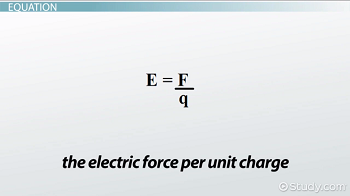The second equation tells you the electric field that a point charge itself creates. A charge q will create its own electric field. And the electric field strength will be stronger the closer you are to it. In this equation, E is the electric field strength again, q is the size of the charge creating the field this time, epsilon-zero is a constant that is always equal to 8.85 x 10^-12, and r is the distance you are away from that charge q.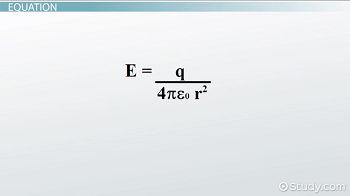So by using the second equation, we can figure out what electric field charges create. And we can use the first equation to figure out the force a second charge will feel when placed inside that electric field, or any electric field for that matter.

## Example Calculation

Okay, let's go through an example. Charge A has a magnitude of 3 x 10^-19 coulombs and creates a magnetic field E. What would be the electric field strength at a distance 5 x 10^-6 meters from charge A? And if you place charge B (5 x 10^-19 coulombs) at this position, what force would it feel?

To unlock this lesson you must be a Study.com Member.

### Register to view this lesson

Are you a student or a teacher?

### Unlock Your Education

#### See for yourself why 30 million people use Study.com

##### Become a Study.com member and start learning now.
Back
What teachers are saying about Study.com

### Earning College Credit

Did you know… We have over 160 college courses that prepare you to earn credit by exam that is accepted by over 1,500 colleges and universities. You can test out of the first two years of college and save thousands off your degree. Anyone can earn credit-by-exam regardless of age or education level.